This topic describes how to calculate the fees for experiments that are run in Machine Learning Studio or Machine Learning Designer. In the following example, the Probabilistic Linear Discriminant Analysis (PLDA) component is used to demonstrate how to calculate the fees.

## Background information

An experiment in Machine Learning Studio or Machine Learning Designer consists of more than one algorithm component, whereas an algorithm component is composed of multiple subtasks. To calculate the fees for an experiment, you need to first calculate the costs of subtasks in each algorithm component, then sum up the fees of all components used in the experiment.

## Procedure

1. Determine the category of an algorithm component.
1. Log on to the Machine Learning Platform for AI (PAI) console.
2. In the left-side navigation pane, click AI Workspaces. On the AI Workspaces page, click the name of the workspace that you want to use.
3. In the left-side navigation pane, choose Model Training > Visualized Modeling (Designer).
4. Optional:In the upper-right corner of the Visualized Modeling (Machine Learning Designer) page, click Old Version(Studio).
5. On the Pipeline list page, select a pipeline and click Enter pipeline.
Note Skip this step if you have performed the previous step to go to Machine Learning Studio.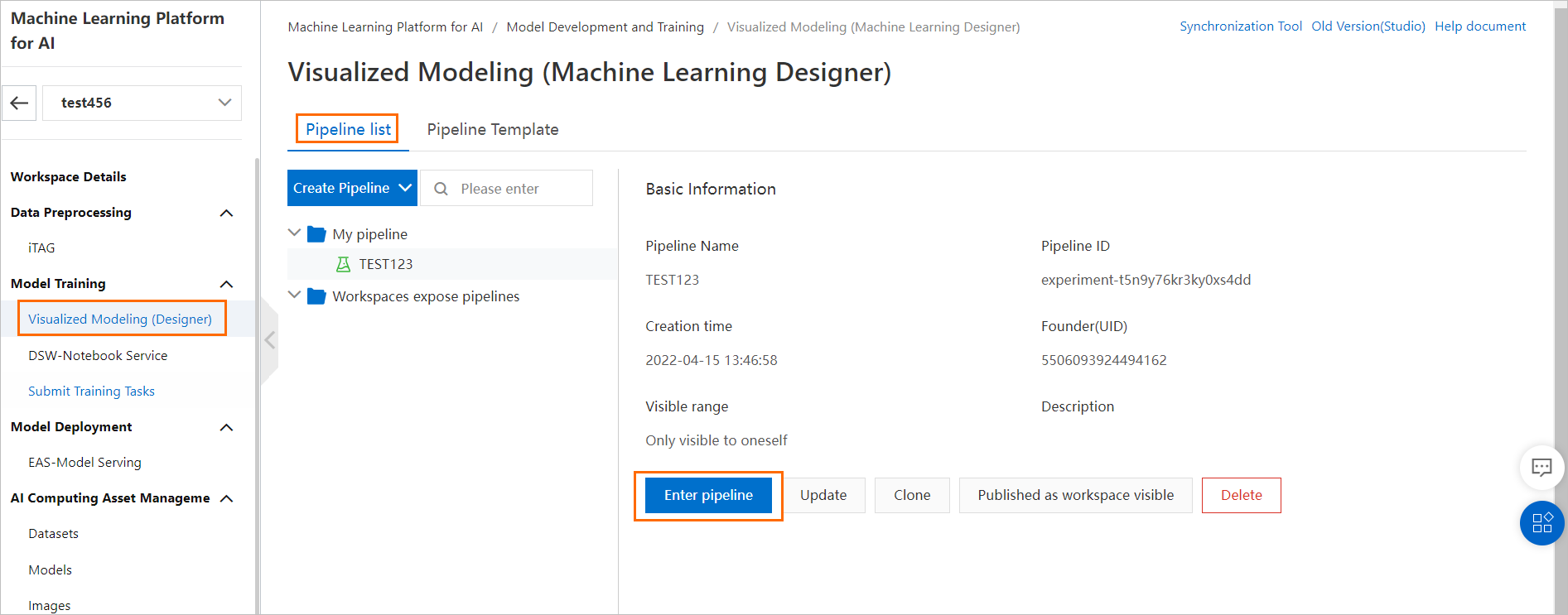6. In the component list, find the PLDA component. The PLDA component belongs to the category of text analysis. The component is billed at a price of USD 0.27 per billable hour.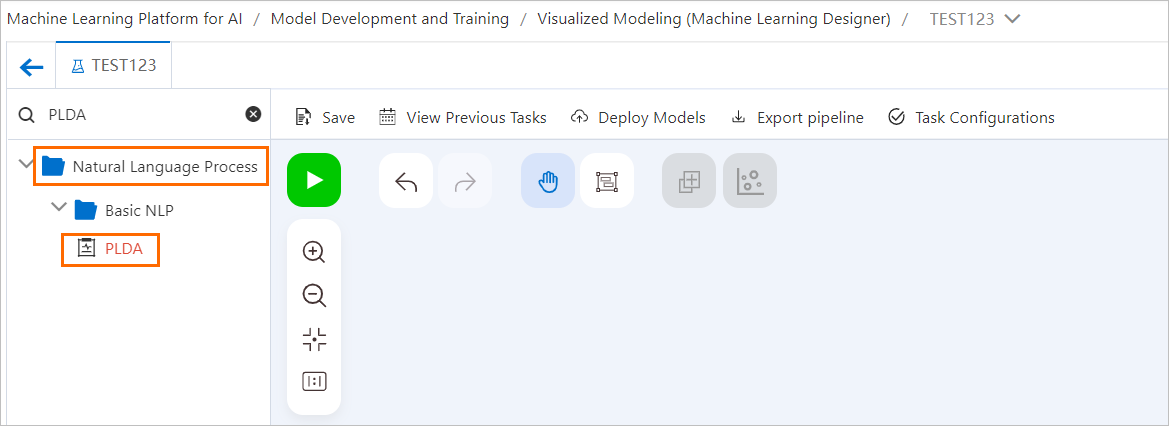2. View the resources consumed for running all jobs of a subtask.
1. On the canvas, right-click the PLDA component.
2. In the menu that appears, click View Log.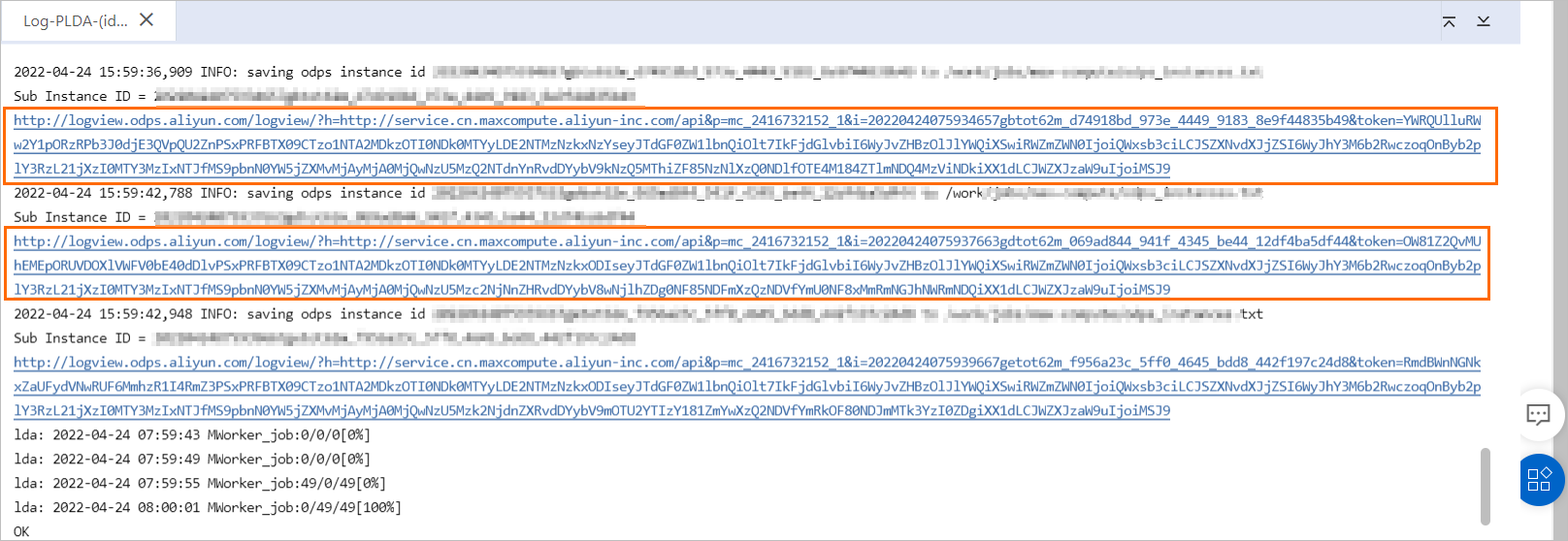4. On the LogView page, click the SourceXML tab.
5. In the TaskPlan section, view the value of the CPU and Memory fields.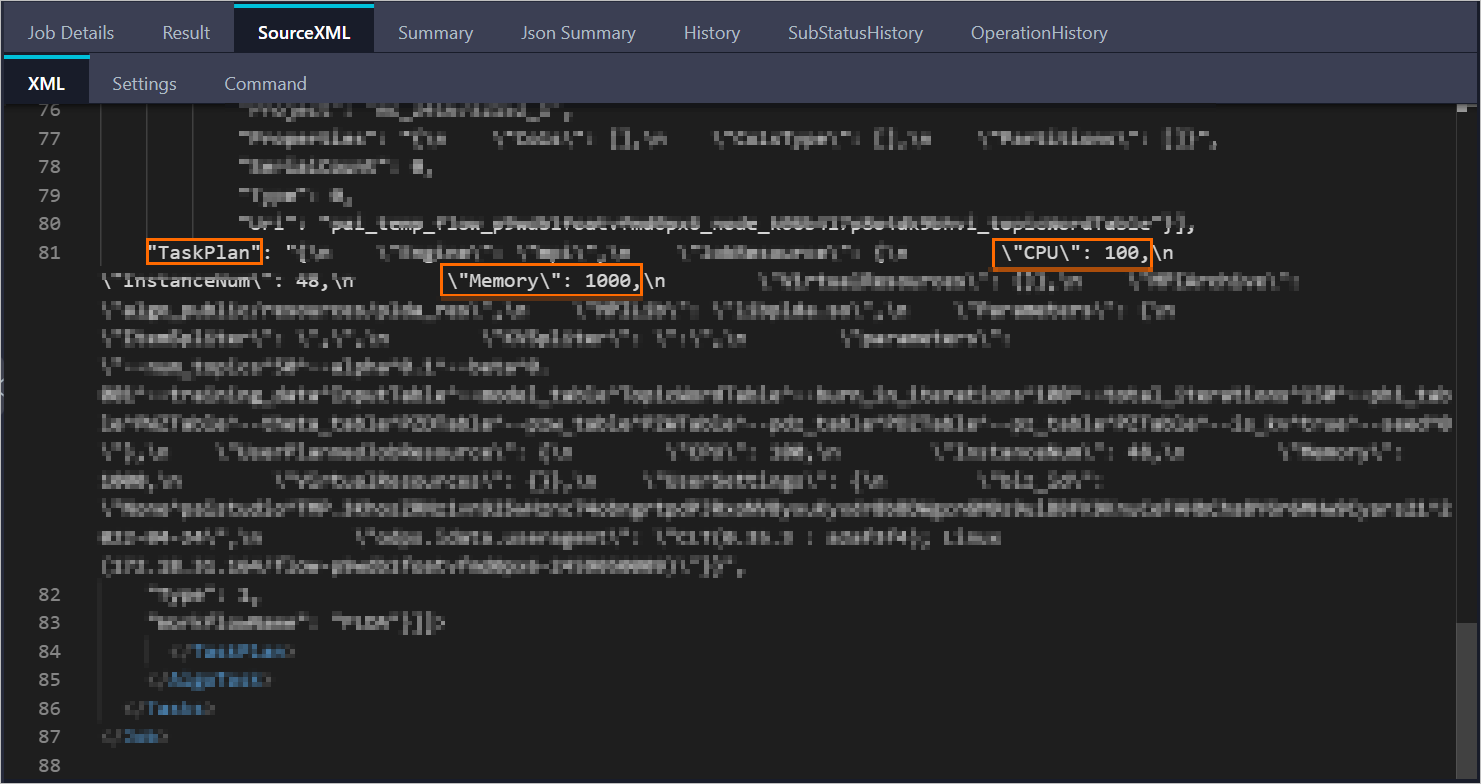• The number of used CPU cores is calculated by dividing the value of the CPU field by 100. In this example, 1 CPU core is used for running the job.
• The unit of the Memory field is MB. In this example, 1 GB of memory is used for running the job.
6. On the LogView page, click the Job Details tab.
7. Click the task object on the AlgoTask_0_0 tab. In the section that appears, click the Terminated tab. The Latency field indicates the operation duration of each job.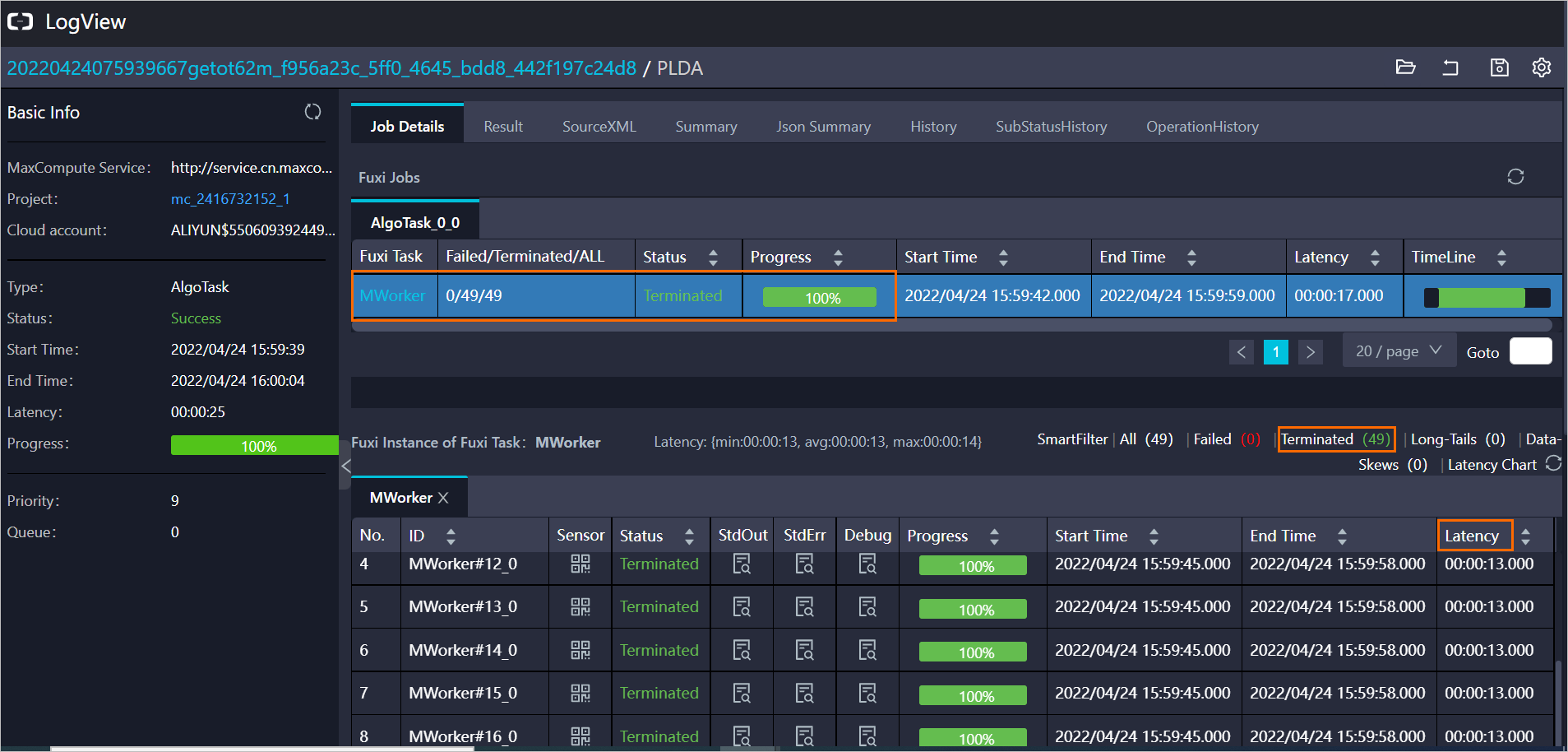In this example, the subtask has 49 jobs, and each job runs for about 13 seconds.
3. Calculate the fee of the subtask.
1. Calculate the number of billable hours used in the subtask. For more information about how to calculate the number of billable hours, see Billing of Machine Learning Studio or Machine Learning Designer.
``Number of billable hours used in the subtask = Max (Number of CPU cores × Usage duration, Memory size × Usage duration/4) = Max [49 × 1 × (26/3,600), 49 × 1 × 26/3,600/4] ≈ 0.35 billable hours``
``Subtask fee = Number of billable hours × Unit price ≈ 0.35 × 0.27 = USD 0.095``